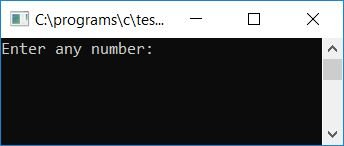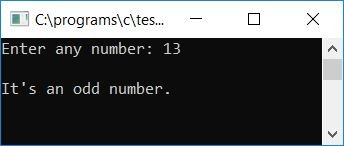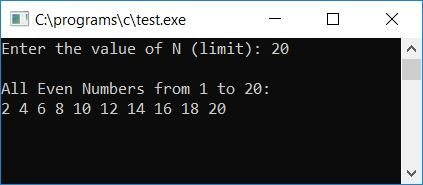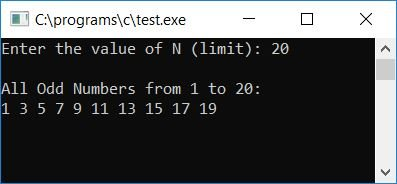# C Program to Check an Even or Odd Number

In this post, we will learn how to create a program that will ask the user to enter any number as input and then check whether it is an even or an odd number. We will also learn how to write a program that will print all the even and odd numbers from the beginning to the end (as specified by the user).

## In C, check whether the numbers are even or odd

In C programming, to determine whether a given number is even or odd, you must ask the user to enter a number to check for even/odd using the divisibility test by 2. That is, if the given number is divisible by 2, then it will be an even number; otherwise, it will be an odd number.

```#include<stdio.h>
#include<conio.h>
int main()
{
int num;
printf("Enter any number: ");
scanf("%d", &num);
if(num%2 == 0)
printf("\nIt's an even number.");
else
printf("\nIt's an odd number.");
getch();
return 0;
}```

Because the preceding program was written and run using the Code::Blocks IDE, you will receive the following output after a successful build and run. This is the first snapshot of the sample run:Now supply any number, say 13, and press the ENTER key to see the output as shown here in the second snapshot of the sample run:Here are some of the main steps used in the above program:

• Receive any number as input, say 13.
• Now, using the if statement, check whether the given number is divisible by 2 without leaving any remainder or not.
• If there is no remainder, then the given number is an even number.
• Otherwise, it will be an odd number.

### Print all even numbers up to N

Here is another program that will ask the user to enter the value of N. Then print all of the even numbers beginning with N. Let's suppose that if the user has supplied 20 as the value of N, then all the even numbers will be printed between 1 and 20, including both.

```#include<stdio.h>
#include<conio.h>
int main()
{
int N, i;
printf("Enter the value of N (limit): ");
scanf("%d", &N);
printf("\nAll Even Numbers from 1 to %d:\n", N);
for(i=1; i<=N; i++)
{
if(i%2 == 0)
printf("%d ", i);
}
getch();
return 0;
}```

Here is the final snapshot of the sample run of the above program:### Print all odd numbers up to N

This program is similar to the previous program. But this time, instead of even numbers, odd numbers get printed as output.

```#include<stdio.h>
#include<conio.h>
int main()
{
int N, i;
printf("Enter the value of N (limit): ");
scanf("%d", &N);
printf("\nAll Odd Numbers from 1 to %d:\n", N);
for(i=1; i<=N; i++)
{
if(i%2 != 0)
printf("%d ", i);
}
getch();
return 0;
}```

This is the final snapshot of the sample run:#### The same program in different languages

C Quiz

« Previous Program Next Program »